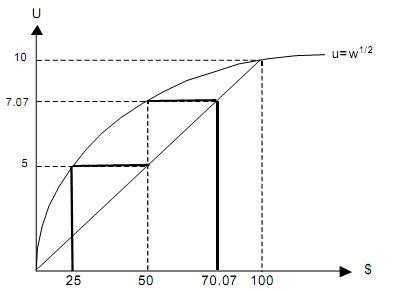#### Attitudes toward Risktaking

Attitudes toward Risktaking:

The rankings were different in each case and they obviously depend on the shape of the utility function over certain sums of money (u(Y)). Note also that lottery 1 is the least risky and 3 the riskiest, because the probability of winning the prize is highest and lowest, respectively, in these cases. In case 1, the consumer is indifferent between taking a risk, or not, and is said to be risk−neutral; in case 2 the consumer always prefer the less risky alternative, and is said to be risk−averse; in case 3 the consumer actually prefers the riskiest alternative and is therefore called a “risk lover”. Figure below shows case 2.

Two important areas of application of the theory of choice under uncertainty are to portfolio problems in financial theory and to the demand for insurance contracts. In financial theory it is an important question to derive prices of uncertain (or risky) asset relative to risk-free assets. In general, if most investors are risk-averse, risky assets will be sold at a lower price than risk-free ones (given that they pay out the same expected sum in the future), or, equivalently, investors demand a risk−premium to invest in such assets.Example:

Let’s assume that we introduce a fourth lottery (L0), where you always win 50, hence it is a risk-free alternative. The utility of this lottery (for the case of a risk-averse consumer) is: U2(L0) = √50 = 7.071. How much extra (in expected value) does this consumer demand in order to accept lottery L1 over L0? In the example above L1 gave the utility: U2(L1) = 5. If L1 is to be as attractive as L0 we must change the probability of getting the high outcome (π) such that,

U2 (L1) = π . √100 + (1 − π) .√0 = 7.071,

with the solution, π = 0.7071, and an expected value of, 0.7071 x 100 +(1 − 0.7071) x 0 = 70.71. The difference, 70.71 − 50 = 20.71, is called a compensatory risk premium.

Latest technology based Economics Online Tutoring Assistance

Tutors, at the www.tutorsglobe.com, take pledge to provide full satisfaction and assurance in Intermediate Microeconomics homework help via online tutoring. Students are getting 100% satisfaction by online tutors across the globe. Here you can get homework help for Intermediate Microeconomics, project ideas and tutorials. We provide email based Intermediate Microeconomics homework help. You can join us to ask queries 24x7 with live, experienced and qualified online tutors specialized in Intermediate Microeconomics. Through Online Tutoring, you would be able to complete your homework or assignments at your home. Tutors at the TutorsGlobe are committed to provide the best quality online tutoring assistance for Economics homework help and assignment help services. They use their experience, as they have solved thousands of the Computer assignments, which may help you to solve your complex issues of Intermediate Microeconomics. TutorsGlobe assure for the best quality compliance to your homework. Compromise with quality is not in our dictionary. If we feel that we are not able to provide the homework help as per the deadline or given instruction by the student, we refund the money of the student without any delay.